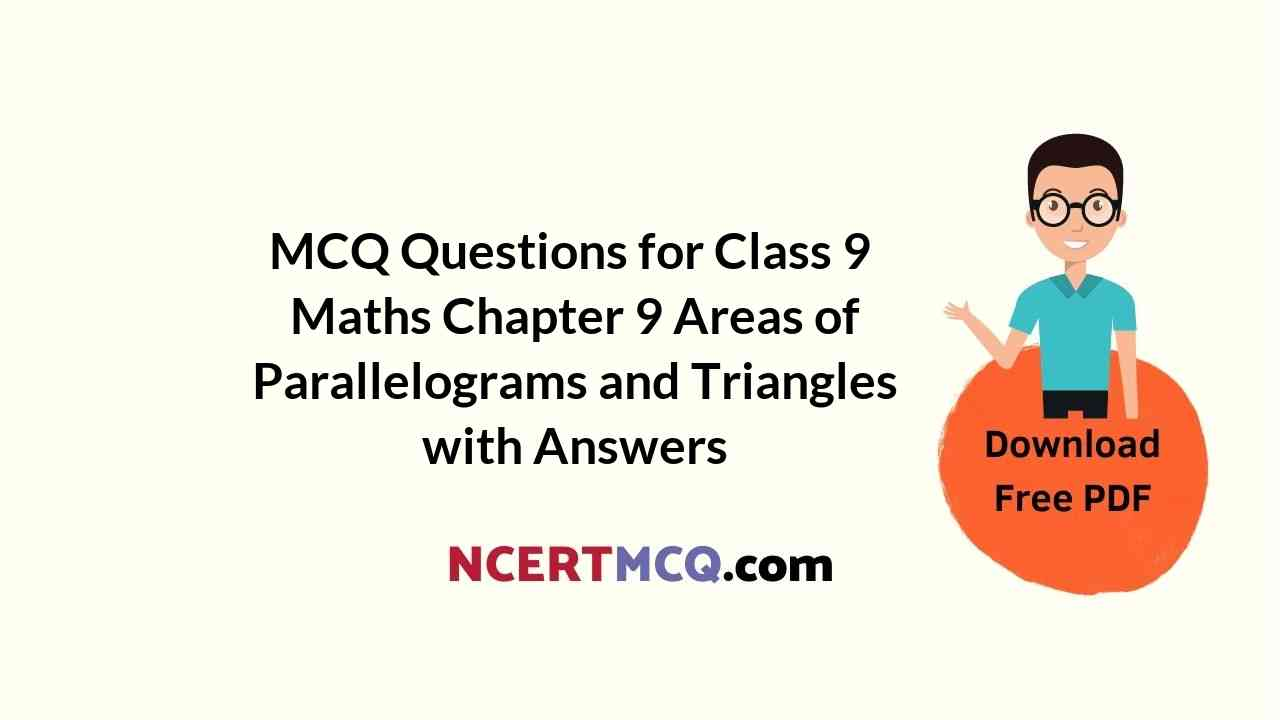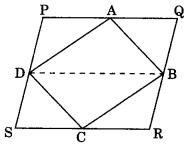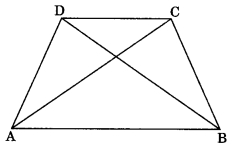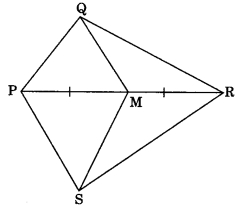Check the below NCERT MCQ Questions for Class 9 Maths Chapter 9 Areas of Parallelograms and Triangles with Answers Pdf free download. MCQ Questions for Class 9 Maths with Answers were prepared based on the latest exam pattern. We have provided Areas of Parallelograms and Triangles Class 10 Maths MCQs Questions with Answers to help students understand the concept very well. https://ncertmcq.com/mcq-questions-for-class-9-maths-with-answers/

Students can also refer to NCERT Solutions for Class 9 Maths Chapter 9 Areas of Parallelograms and Triangles for better exam preparation and score more marks.

## Areas of Parallelograms and Triangles Class 9 MCQs Questions with Answers

Class 9 Maths Chapter 9 MCQ Question 1.
Two parallelograms are on equal bases and between the same parallels. The ratio of their areas is
(a) 1 : 2
(b) 1 : 1
(c) 2 : 1
(d) 3 : 1

Area Of Parallelogram And Triangle Class 9 MCQ Question 2.
ABCD is a quadrilateral whose diagonal AC divides it in two parts of equal area, then ABCD is a
(a) rectangle
(b) rhombus
(c) parallelogram
(d) need not be any of (a), (b) or (c)

Answer: (d) need not be any of (a), (b) or (c)

MCQ On Areas Of Parallelograms And Triangles Class 9 Question 3.
If a triangle and a parallelogram are on the same base and between same parallels, then the ratio of the area of the triangle to the area of parallelogram is
(a) 1 : 3
(b) 1 : 2
(c) 3 : 1
(d) 1 : 4

Class 9 Maths Chapter 9 MCQ With Answers Question 4.
The median of a triangle divides it into two
(a) isosceles triangle
(b) congruent triangles
(c) right angled triangle
(d) triangles of equal areas

Answer: (d) triangles of equal areas

MCQ Questions For Class 9 Maths Ncert Chapter 9 Question 5.
PQRS is a parallelogram and A and B are any points on PQ and QR. If ar(PQRS) = 48 cm², then ar(ΔPBS) + ar(ΔASR) is equal to(a) 96 cm²
(b) 36 cm²
(c) 48 cm²
(d) 24 cm²

Class 9 Maths Ch 9 MCQ Question 6.
A, B, C and D are the mid-points of sides of parallelogram PQRS. If ar(PQRS) = 36 cm², then ar(ABCD) is(a) 24 cm²
(b) 18 cm²
(c) 30 cm²
(d) 36 cm²

Areas Of Parallelograms And Triangles Class 9 MCQ Question 7.
ABCD is a trapezium in which AB || DC. If ar(ΔABD) = 24 cm² and AB = 8 cm, then height of ΔABC is(a) 3 cm
(b) 6 cm
(c) 8 cm
(d) 4 cm

Area Of Triangle And Quadrilateral Class 9 MCQ Question 8.
PQRS is a parallelogram. If X and Y are the mid-points of PQ and SR and diagonal SQ is joined, then ar(XQRY) : ar(ΔQSR) is(a) 1 : 2
(b) 1 : 4
(c) 1 : 1
(d) 2 : 1

MCQ Questions For Class 9 Maths Areas Of Parallelograms And Triangles Question 9.
In quadrilateral PQRS, M is the mid-point of PR. If ar(SMQR) = 18 cm², then ar(PQMS) is(a) 24 cm²
(b) 12 cm²
(c) 18 cm²
(d) 36 cm²

Class 9 Maths Chapter 9 MCQ Online Test Question 10.
D and E are the mid-points of BC and AD respectively. If ar(ΔABC) = 12 cm², then ar(ΔBDE) is
(a) 5 cm²
(b) 6 cm²
(c) 3 cm²
(d) 9 cm²